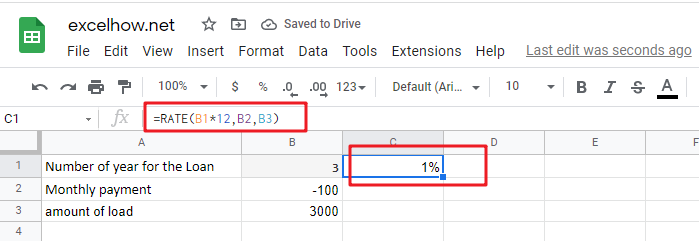# ExcelHow

This post will guide you how to use Google Sheets RATE function with syntax and examples in google spreadsheet .

## Description

The Google Sheets RATE function returns the interest rate per payment period of an annuity.

The RATE function is a build-in function in Google Sheets and it is categorized as a Financial Function.

## Syntax

The syntax of the RATE function is as below:

`= RATE(number_of_periods, payment_per_period, present_value, [future_value, end_or_beginning, rate_guess])`

Where the RATE function arguments are:

• `number_of_periods` -This is a required argument. The total number of payments periods in an annuity.
• `payment_per_period` – This is a required argument. The amount of the payment made each period.
• `present_value` – This is a required argument. The present value of the payments.
• `future_value` – This is an optional argument. The future value or a cash balance you want to attain after the last payment is made. If fv is omitted, it is assumed to be 0 (zero), that is, the future value of a loan is 0.
• `end_or_beginning` – This is an optional argument. The number 0 (zero) or 1 and indicates when payments are due.
 Set type equal to If payments are due 0 or omitted At the end of the period 1 At the beginning of the period
• `Guess` – This is an optional argument. An initial guess at the internal rate of return. if it is omitted, and it will be set as 0.1 or 10%.

Note:

• Make sure that you are consistent about the units you use for specifying rate and nper. If you make monthly payments on a four-year loan at an annual interest rate of 12 percent, use 12%/12 for rate and 4*12 for number_of_periods. If you make annual payments on the same loan, use 12 percent for rate and 4 for number_of_periods.

## Google Sheets RATE Function Examples

The below examples will show you how to use Google Sheets RATE Function to calculate the interest rate per period of an annuity.

#1 to get the monthly rate of the loan, using the following formula:

`=RATE(B1*12,B2,B3)`